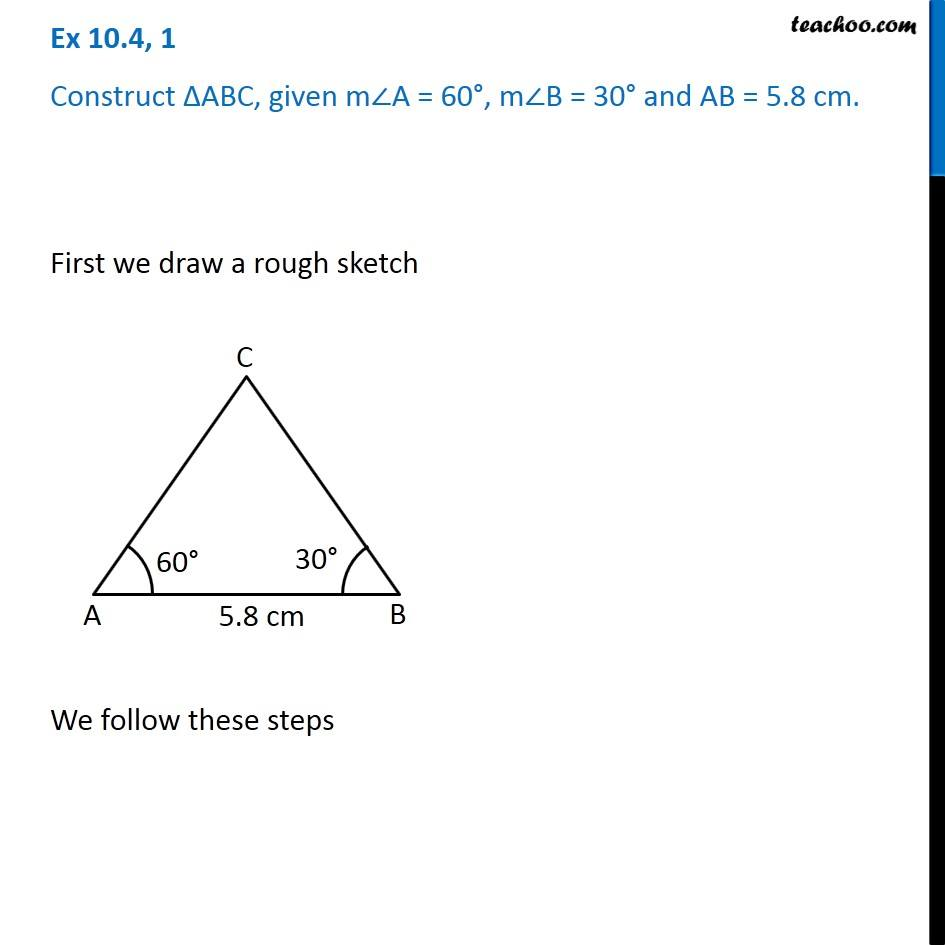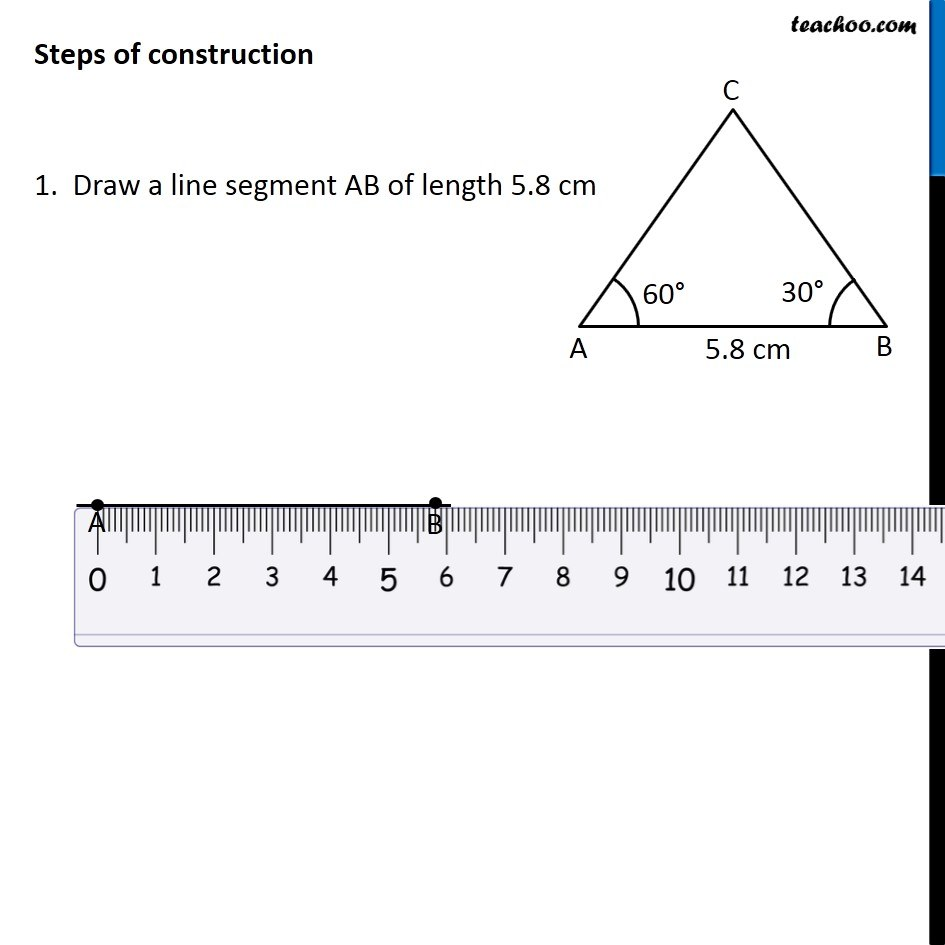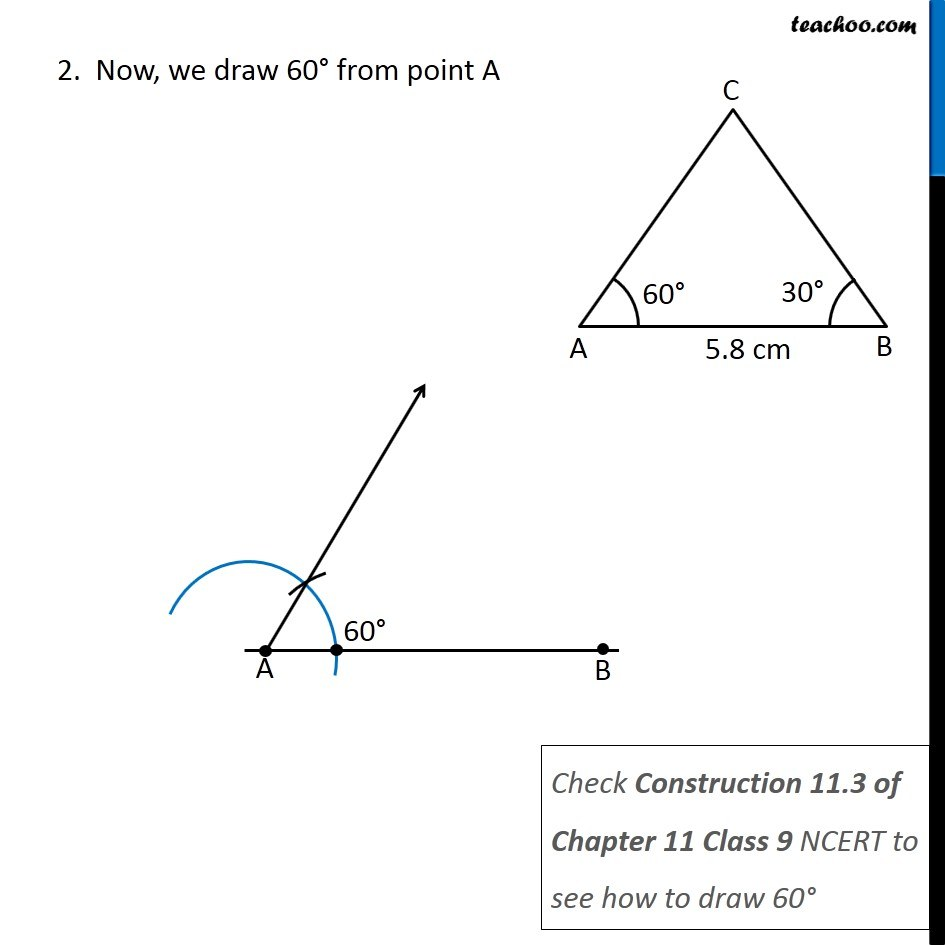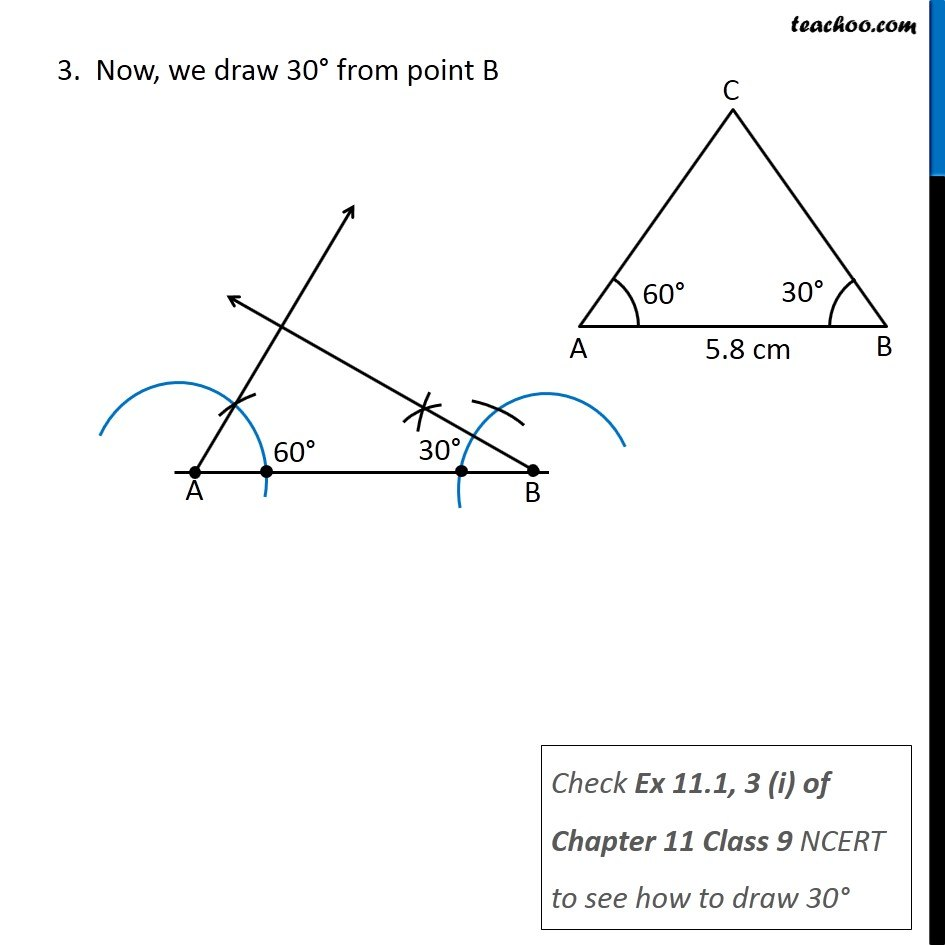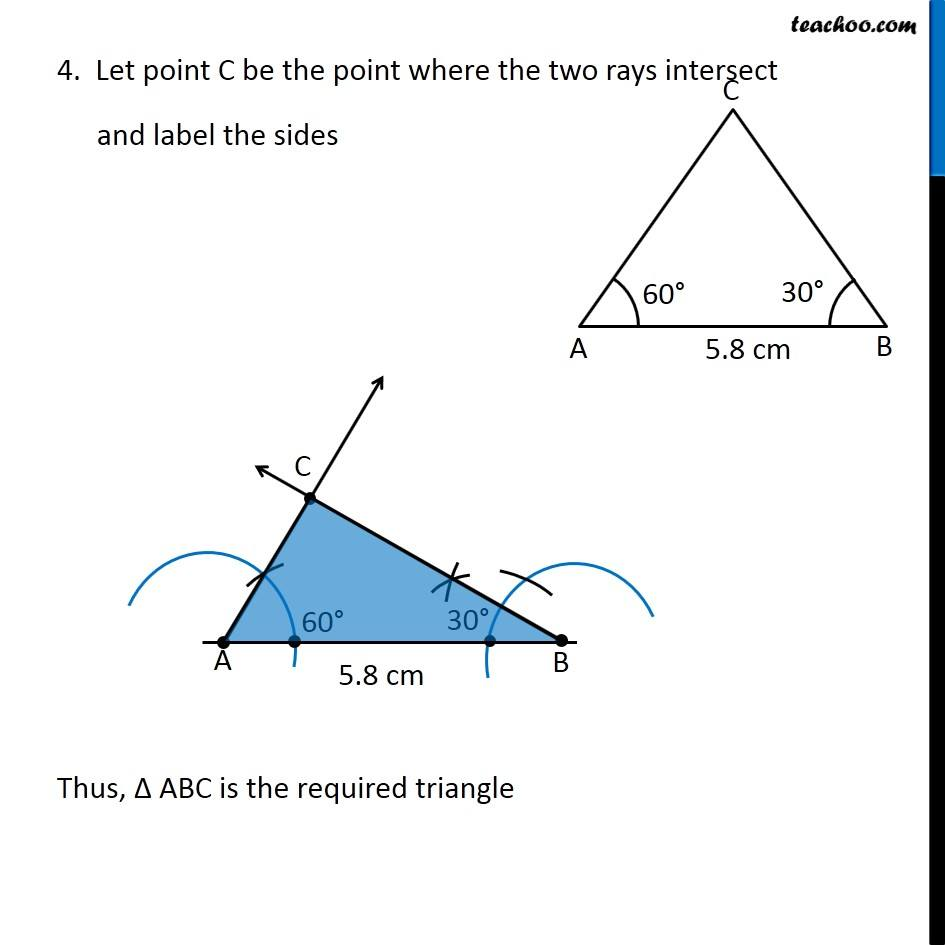1. Chapter 10 Class 7 Practical Geometry
2. Serial order wise
3. Ex 10.4

Transcript

Ex 10.4, 1 Construct ∆ABC, given m∠A = 60°, m∠B = 30° and AB = 5.8 cm. First we draw a rough sketch We follow these steps Steps of construction 1. Draw a line segment AB of length 5.8 cm 2. Now, we draw 60° from point A Check Construction 11.3 of Chapter 11 Class 9 NCERT to see how to draw 60° 3. Now, we draw 30° from point B Check Ex 11.1, 3 (i) of Chapter 11 Class 9 NCERT to see how to draw 30° 4. Let point C be the point where the two rays intersect and label the sides

Ex 10.4